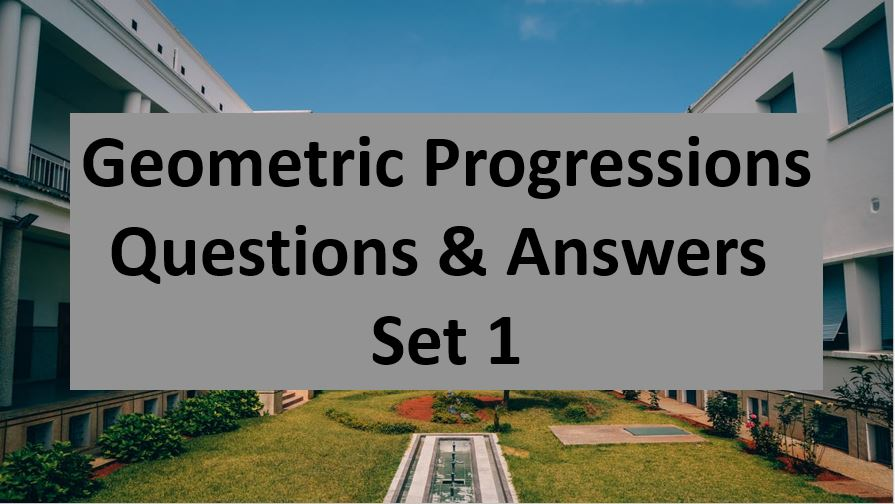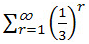Friday, October 22, 2021
Home > Question and Answer > Geometric Progression Question And Answer Set 1

# Geometric Progression Question And Answer Set 1# Geometric Progression Question and Answer Set 1

Hi students, welcome to Amans Maths Blogs (AMB). On this post, you will get the Geometric Progression Question and Answer Set 1 is the collection of some important questions. Practice these questions for SSC CGL CHSL CAT NTSE exams etc.. It will help you to practice the questions on the topics of maths as geometric progression based questions of algebra.

Geometric Progression Question and Answer Set 1: Ques No 1

The value ofis

Options:

A. 1

B. 1/2

C. 1/3

D. Infinite

Geometric Progression Question and Answer Set 1: Ques No 2

In a geometric progression, the common ratio is half of the first term. If 4th term of the GP is 32, then the value of 15th term is

Options:

A. 24

B. 28

C. 216

D. None of these

Geometric Progression Question and Answer Set 1: Ques No 3

The sum of n term of the series 0.8 + 0.88 + 0.888 + 0.8888 + … is

Options:

A. 0.8n

B. 8n-1

C. (8/81)(10-n – 9n + 1)

D. (8/81)(10-n + 9n – 1)

Geometric Progression Question and Answer Set 1: Ques No 4

The sum of n term of the GP 7/10 + 7/100 + 7/1000 + 7/10000 + … is

Options:

A. (7/9)(10-n – 1)

B. (7/81)(10-n – 1)

C. (9/7)(10-n – 1)

D. (7/90)(10-n – 1)

Geometric Progression Question and Answer Set 1: Ques No 5

If (xa+2 + ya+2)/(xa+1 + ya+2) is the GM between x and y, then the value of a is

Options:

A. -2/3

B. -1/4

C. -3/2

D. 7/6

Geometric Progression Question and Answer Set 1: Ques No 6

In a GP, the sum of the first two terms is 36 and the product of the first and third terms is 9 times the second term. The sum of the first 8 terms is

Options:

A. 3480/81

B. 3280/81

C. 3680/81

D. 3880/81

Geometric Progression Question and Answer Set 1: Ques No 7

There are five numbers are in AP with common difference is not equal to zero. If the first, third and fourth of these numbers are in GP, then which of the following is true?

Options:

A. First number is zero.

B. Third number is zero.

C. Fifth number is zero.

D. None of these

AMBiPi
error: Content is protected !!